# Control Flow IV Control Flow Optimizations Start Dataflow

• Slides: 29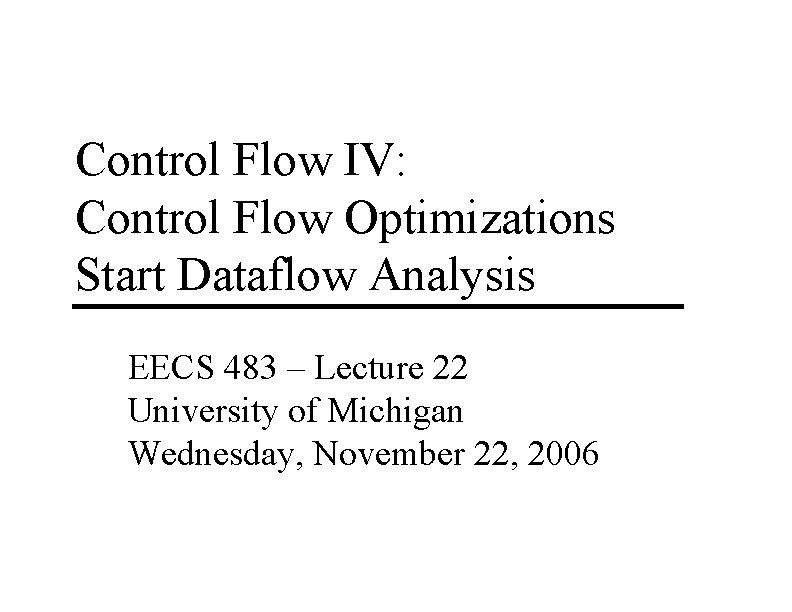Control Flow IV: Control Flow Optimizations Start Dataflow Analysis EECS 483 – Lecture 22 University of Michigan Wednesday, November 22, 2006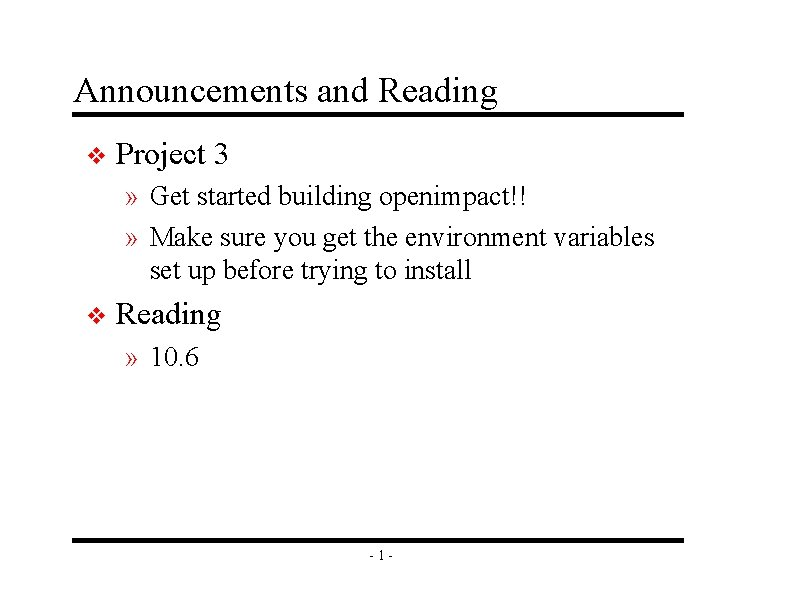Announcements and Reading v Project 3 » Get started building openimpact!! » Make sure you get the environment variables set up before trying to install v Reading » 10. 6 -1 -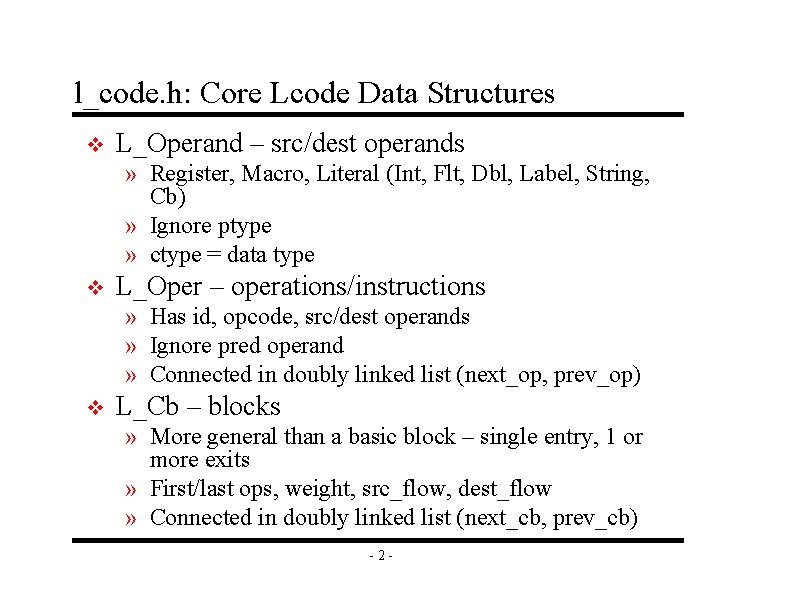l_code. h: Core Lcode Data Structures v L_Operand – src/dest operands » Register, Macro, Literal (Int, Flt, Dbl, Label, String, Cb) » Ignore ptype » ctype = data type v L_Oper – operations/instructions » Has id, opcode, src/dest operands » Ignore pred operand » Connected in doubly linked list (next_op, prev_op) v L_Cb – blocks » More general than a basic block – single entry, 1 or more exits » First/last ops, weight, src_flow, dest_flow » Connected in doubly linked list (next_cb, prev_cb) -2 -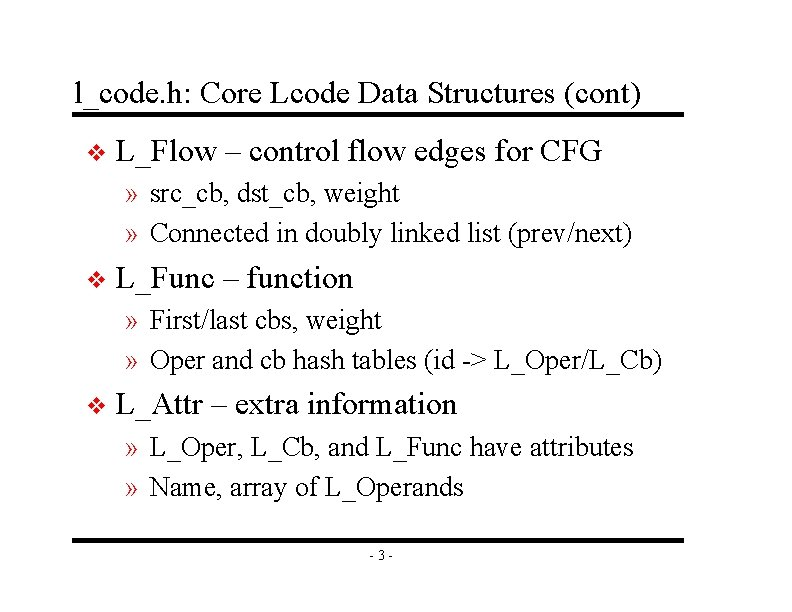l_code. h: Core Lcode Data Structures (cont) v L_Flow – control flow edges for CFG » src_cb, dst_cb, weight » Connected in doubly linked list (prev/next) v L_Func – function » First/last cbs, weight » Oper and cb hash tables (id -> L_Oper/L_Cb) v L_Attr – extra information » L_Oper, L_Cb, and L_Func have attributes » Name, array of L_Operands -3 -Example Opti: Local Constant Propagation for (op. A = cb->first_op; op. A != NULL; op. A = op. A->next_op) { /* Match pattern */ if (!L_move_opcode (op. A)) continue; if (!L_is_constant (op. A->src)) continue; for (op. B = op. A->next_op; op. B != NULL; op. B = op. B->next_op) { if (!L_is_src_operand (op. A->dest, op. B)) continue; if (!L_can_change_src_operand (op. B, op. A->dest)) continue; if (!L_no_defs_between (op. A->dest, op. A, op. B)) continue; macro_flag = L_is_fragile_macro (op. A->dest); load_flag = 0; store_flag = 0; if (!L_no_danger (macro_flag, load_flag, store_flag, op. A, op. B)) break; /* Replace pattern */ for (i = 0; i < L_max_src_operand; i++) { if (L_same_operand (op. A->dest, op. B->src[i])) { op. B->src[i] = L_copy_operand (op. A->src); } } In reality, more code than this, but this is the -4 - important stuffRunning Things Manually, Debugging v First, generate lc files using script » compile_bench wc –c 2 lc v tar zxvf wc. lc. tgz » These are the unoptimized Lcode files v Run Lopti manually » Lopti –Farch=IMPACT –Fmodel=Lcode –Fopti=1 –i wc. lc –o wc. O Ÿ opti=0: will disable all optimizations » Compare wc. lc and wc. O, should see results of your optimizations » Note, if benchmark has multiple files, need to run Lopti on each. lc file -5 -Running Things Manually, Debugging - cont v Running Lemulate manually » ls *. O > list » Lemulate –i list » Generates a. c file for each. O file v Compile the. c files with gcc » gcc –g *. c \$IMPACT_REL_PATH/platform/x 86 lin_gcc/impact_lemul_lib. o –lm » Generates a. out, so now run it. v Debugging – gdb » Can gdb the benchmark a. out or Lopti » Can also add print statements to Lopti -6 -From Last Time: Loop Unroll Summary v Goals » Reduce number of executed branches inside loop Ÿ Note: Type 1/Type 2 only » Enable the overlapped execution of multiple iterations Ÿ Reorder instructions between iterations » Expose optimizations across iterations v v v Type 1 – compile-time constant trip count Type 2 – counted, but trip count unknown at compile time Type 3 – non-counted -7 -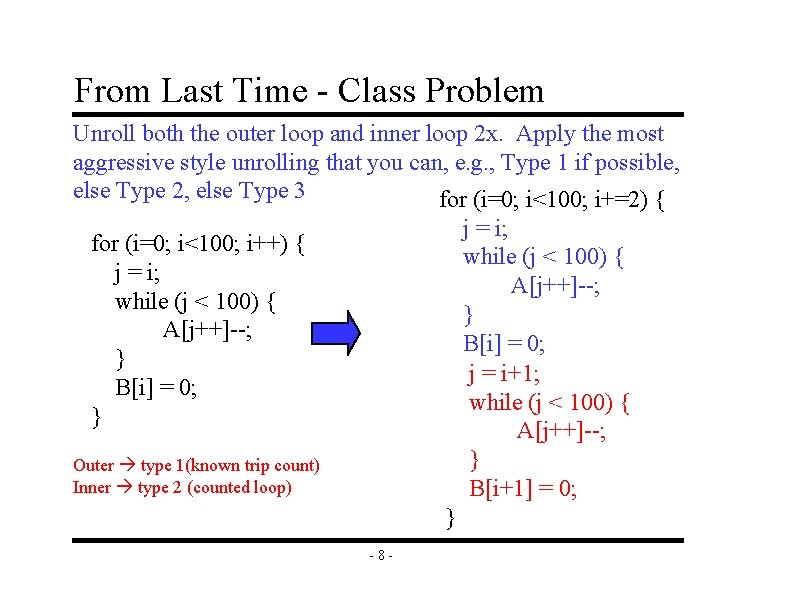From Last Time - Class Problem Unroll both the outer loop and inner loop 2 x. Apply the most aggressive style unrolling that you can, e. g. , Type 1 if possible, else Type 2, else Type 3 for (i=0; i<100; i+=2) { j = i; while (j < 100) { A[j++]--; } B[i] = 0; j = i+1; while (j < 100) { A[j++]--; } B[i+1] = 0; for (i=0; i<100; i++) { j = i; while (j < 100) { A[j++]--; } B[i] = 0; } Outer type 1(known trip count) Inner type 2 (counted loop) } -8 -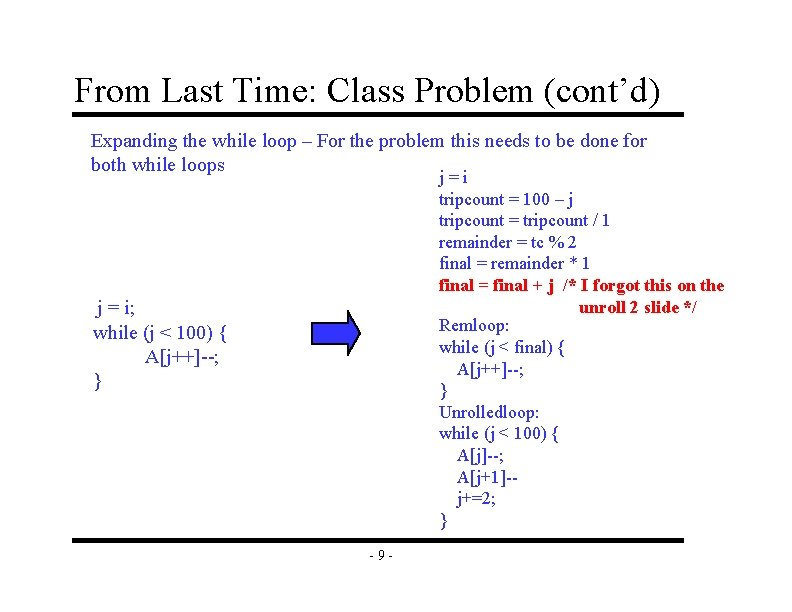From Last Time: Class Problem (cont’d) Expanding the while loop – For the problem this needs to be done for both while loops j=i tripcount = 100 – j tripcount = tripcount / 1 remainder = tc % 2 final = remainder * 1 final = final + j /* I forgot this on the unroll 2 slide */ Remloop: while (j < final) { A[j++]--; } Unrolledloop: while (j < 100) { A[j]--; A[j+1]-j+=2; } j = i; while (j < 100) { A[j++]--; } -9 -Loop Peeling v Unravel first P iterations of a loop » Enable overlap of instructions from the peeled iterations with preheader instructions » Increase potential for ILP » Enables further optimization of main body Preheader Iteration 1 More iters? r 1 = MEM[r 2 + 0] r 4 = r 1 * r 5 r 6 = r 4 << 2 MEM[r 3 + 0] = r 6 r 2 = r 2 + 1 bge r 2 100 Done Loop: r 1 = MEM[r 2 + 0] r 4 = r 1 * r 5 r 6 = r 4 << 2 MEM[r 3 + 0] = r 6 r 2 = r 2 + 1 blt r 2 100 Loop Done: - 10 -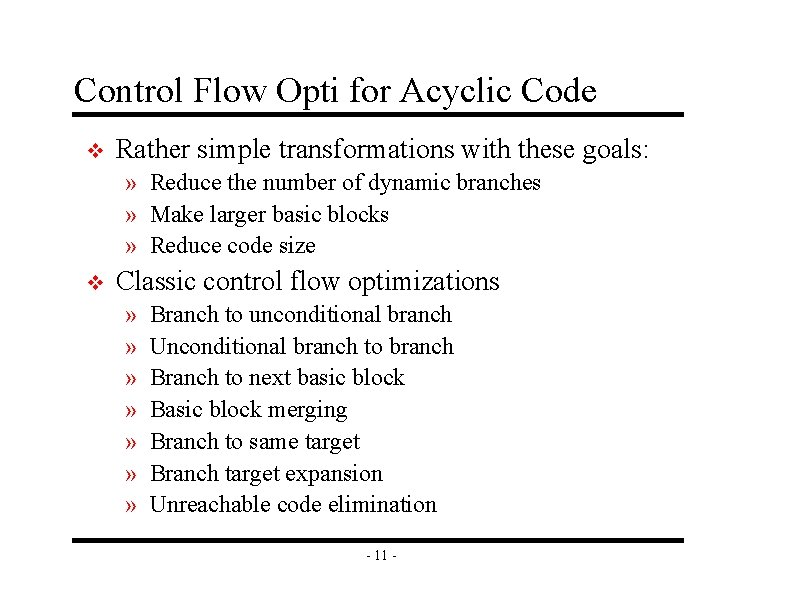Control Flow Opti for Acyclic Code v Rather simple transformations with these goals: » Reduce the number of dynamic branches » Make larger basic blocks » Reduce code size v Classic control flow optimizations » » » » Branch to unconditional branch Unconditional branch to branch Branch to next basic block Basic block merging Branch to same target Branch target expansion Unreachable code elimination - 11 -Acyclic Control Flow Optimizations (1) 1. Branch to unconditional branch L 1: if (a < b) goto L 2. . . L 2: goto L 3 L 1: if (a < b) goto L 3. . . L 2: goto L 3 may be deleted 2. Unconditional branch to branch L 1: if (a < b) goto L 3 goto L 4: L 1: goto L 2. . . L 2: if (a < b) goto L 3 may be deleted L 2: if (a < b) goto L 3 L 4: - 12 -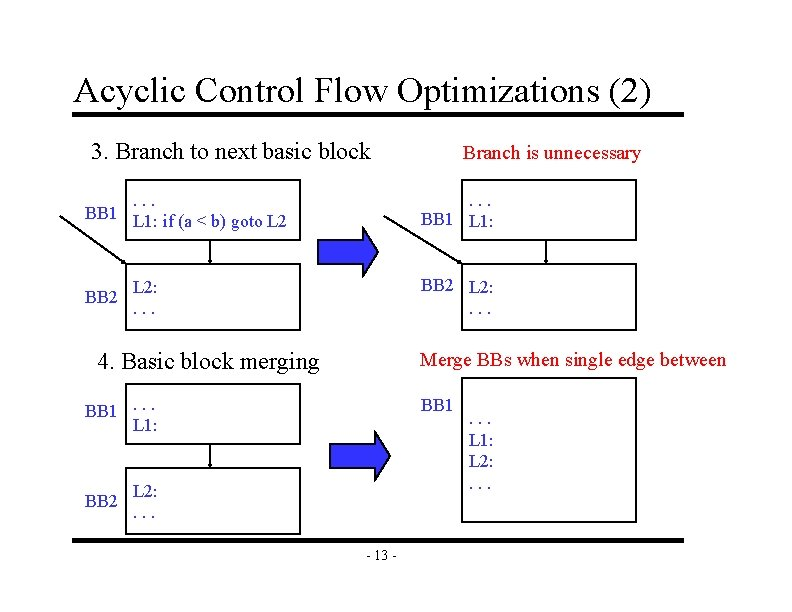Acyclic Control Flow Optimizations (2) 3. Branch to next basic block. . . BB 1 L 1: if (a < b) goto L 2 BB 2 . . . BB 1 L 1: BB 2 L 2: . . . 4. Basic block merging Merge BBs when single edge between BB 1. . . L 1: BB 2 Branch is unnecessary BB 1 L 2: . . . - 13 - . . . L 1: L 2: . . .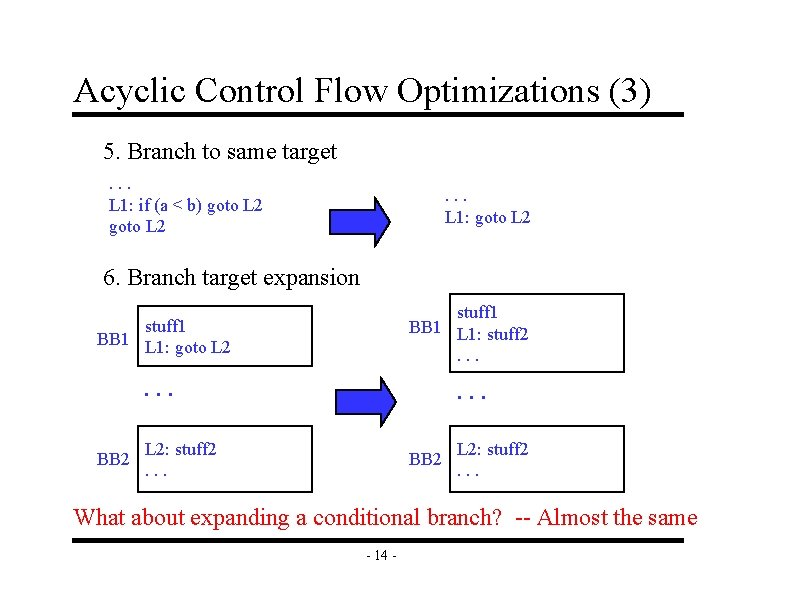Acyclic Control Flow Optimizations (3) 5. Branch to same target. . . L 1: if (a < b) goto L 2 . . . L 1: goto L 2 6. Branch target expansion stuff 1 BB 1 L 1: goto L 2 stuff 1 BB 1 L 1: stuff 2. . BB 2 L 2: stuff 2. . . What about expanding a conditional branch? -- Almost the same - 14 -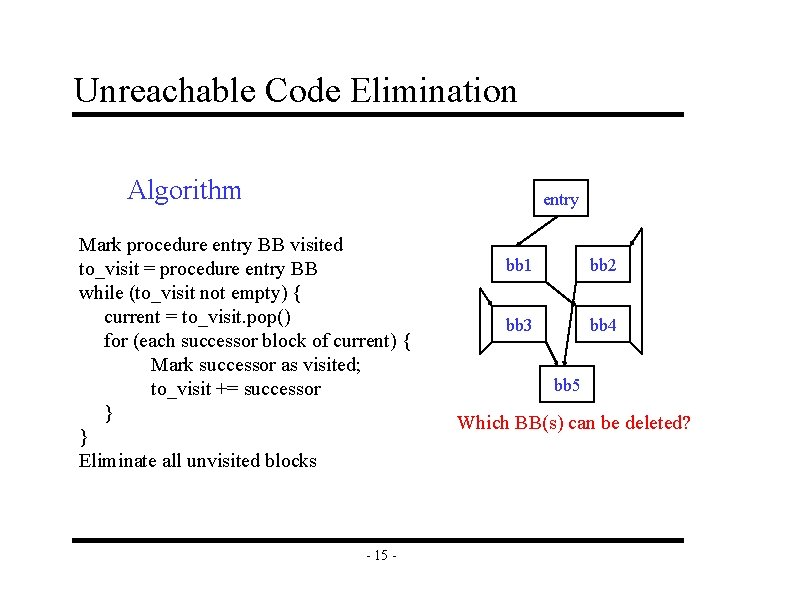Unreachable Code Elimination Algorithm entry Mark procedure entry BB visited to_visit = procedure entry BB while (to_visit not empty) { current = to_visit. pop() for (each successor block of current) { Mark successor as visited; to_visit += successor } } Eliminate all unvisited blocks - 15 - bb 1 bb 2 bb 3 bb 4 bb 5 Which BB(s) can be deleted?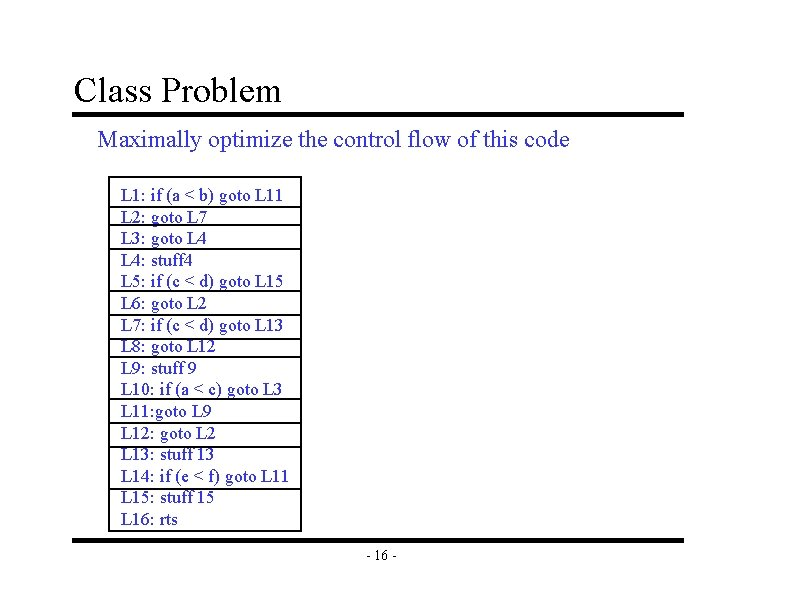Class Problem Maximally optimize the control flow of this code L 1: if (a < b) goto L 11 L 2: goto L 7 L 3: goto L 4: stuff 4 L 5: if (c < d) goto L 15 L 6: goto L 2 L 7: if (c < d) goto L 13 L 8: goto L 12 L 9: stuff 9 L 10: if (a < c) goto L 3 L 11: goto L 9 L 12: goto L 2 L 13: stuff 13 L 14: if (e < f) goto L 11 L 15: stuff 15 L 16: rts - 16 -Profile-based Control Flow Optimization: Trace Selection v Trace - Linear collection of basic blocks that tend to execute in sequence 10 BB 1 90 » “Likely control flow path” » Acyclic (outer backedge ok) v v Side entrance – branch into the middle of a trace Side exit – branch out of the middle of a trace 80 20 BB 2 BB 3 80 20 BB 4 10 BB 5 90 10 BB 6 10 - 17 -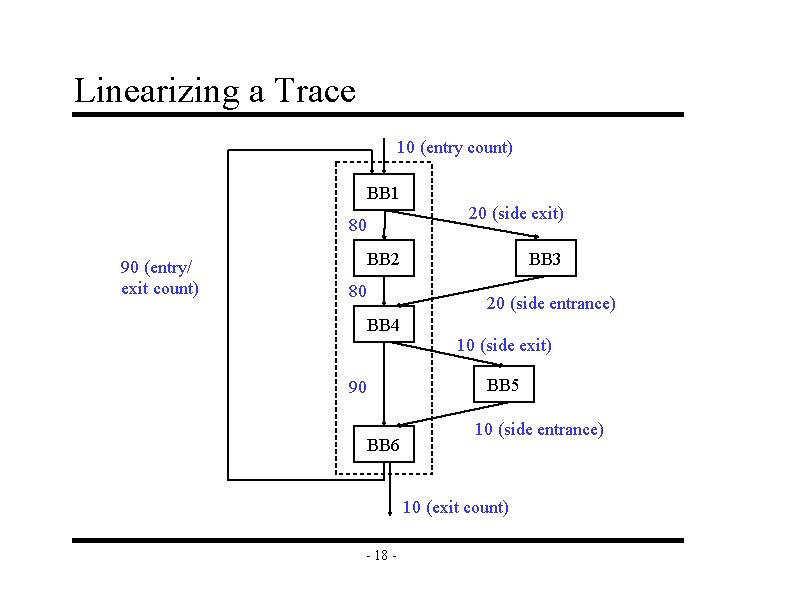Linearizing a Trace 10 (entry count) BB 1 20 (side exit) 80 90 (entry/ exit count) BB 2 80 BB 3 20 (side entrance) BB 4 10 (side exit) BB 5 90 BB 6 10 (side entrance) 10 (exit count) - 18 -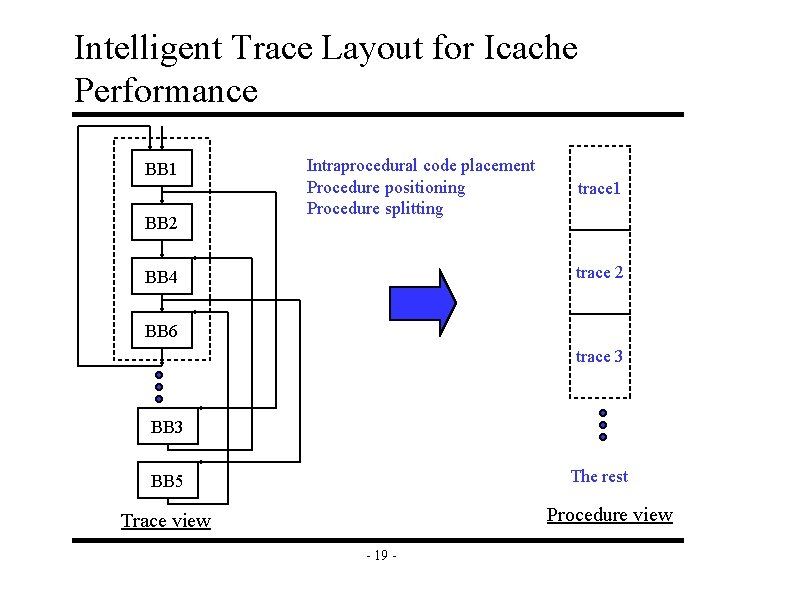Intelligent Trace Layout for Icache Performance BB 1 BB 2 Intraprocedural code placement Procedure positioning Procedure splitting trace 1 trace 2 BB 4 BB 6 trace 3 BB 3 The rest BB 5 Procedure view Trace view - 19 -Dataflow Analysis + Optimization v r 1 = r 2 + r 3 r 6 = r 4 – r 5 Control flow analysis » Treat BB as black box » Just care about branches v Now. . . » Start looking at operations in BBs » What’s computed and where r 4 = 4 r 6 = 8 r 6 = r 2 + r 3 r 7 = r 4 – r 5 v Classical optimizations » Make the computation more efficient » Get rid of redundancy » Simplify v Ex: Common Subexpression Elimination » Is r 2 + r 3 redundant? What about r 4 - r 5? » What if there were 1000 BB’s » Dataflow analysis !! - 20 -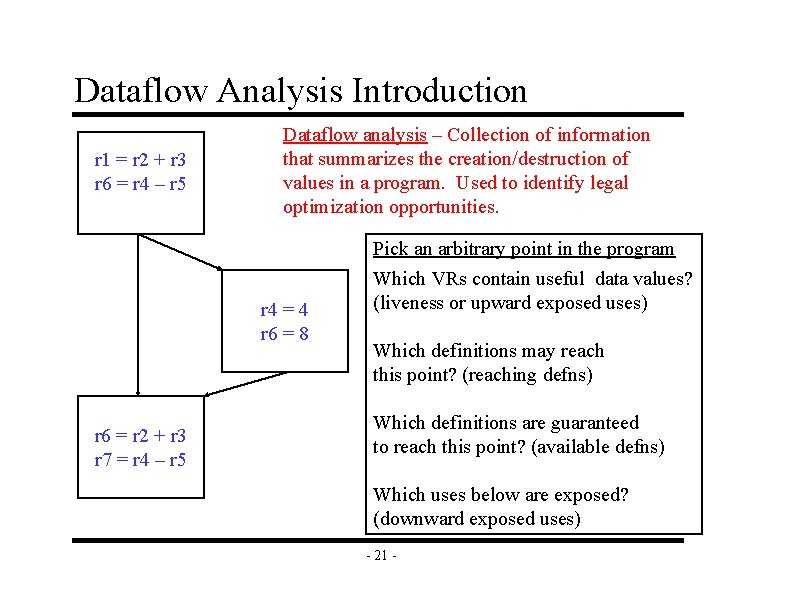Dataflow Analysis Introduction r 1 = r 2 + r 3 r 6 = r 4 – r 5 Dataflow analysis – Collection of information that summarizes the creation/destruction of values in a program. Used to identify legal optimization opportunities. r 4 = 4 r 6 = 8 r 6 = r 2 + r 3 r 7 = r 4 – r 5 Pick an arbitrary point in the program Which VRs contain useful data values? (liveness or upward exposed uses) Which definitions may reach this point? (reaching defns) Which definitions are guaranteed to reach this point? (available defns) Which uses below are exposed? (downward exposed uses) - 21 -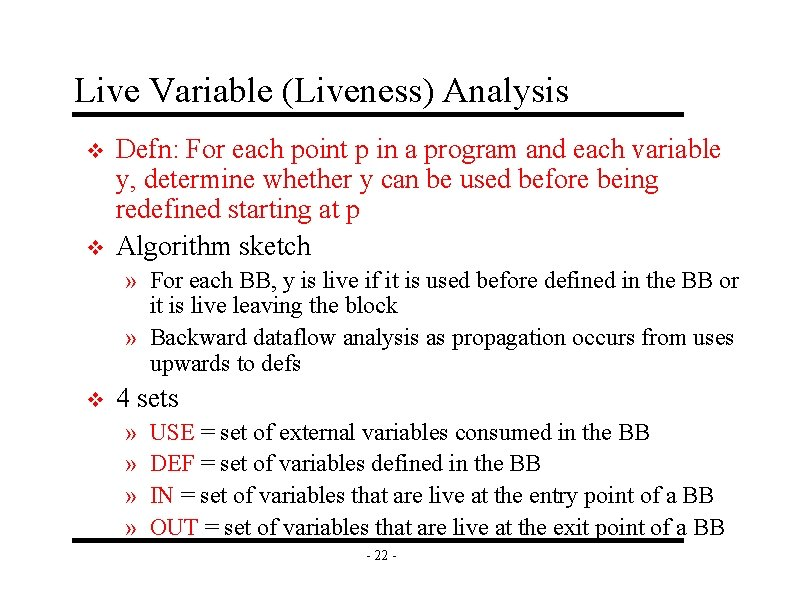Live Variable (Liveness) Analysis v v Defn: For each point p in a program and each variable y, determine whether y can be used before being redefined starting at p Algorithm sketch » For each BB, y is live if it is used before defined in the BB or it is live leaving the block » Backward dataflow analysis as propagation occurs from uses upwards to defs v 4 sets » » USE = set of external variables consumed in the BB DEF = set of variables defined in the BB IN = set of variables that are live at the entry point of a BB OUT = set of variables that are live at the exit point of a BB - 22 -Liveness Example r 2, r 3, r 4, r 5 are all live as they are consumed later, r 6 is dead as it is redefined later r 1 = r 2 + r 3 r 6 = r 4 – r 5 r 4 is dead, as it is redefined. So is r 6. r 2, r 3, r 5 are live r 4 = 4 r 6 = 8 r 6 = r 2 + r 3 r 7 = r 4 – r 5 What does this mean? r 6 = r 4 – r 5 is useless, it produces a dead value !! Get rid of it! - 23 -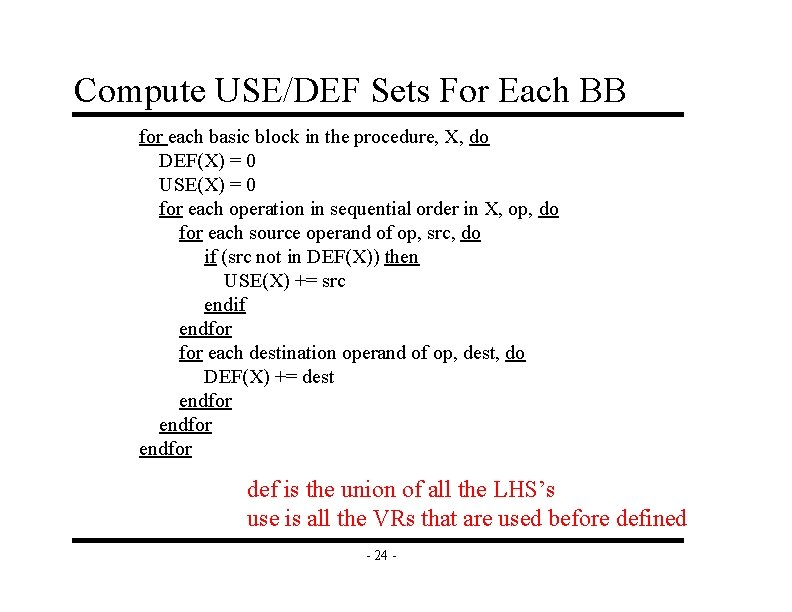Compute USE/DEF Sets For Each BB for each basic block in the procedure, X, do DEF(X) = 0 USE(X) = 0 for each operation in sequential order in X, op, do for each source operand of op, src, do if (src not in DEF(X)) then USE(X) += src endif endfor each destination operand of op, dest, do DEF(X) += dest endfor def is the union of all the LHS’s use is all the VRs that are used before defined - 24 -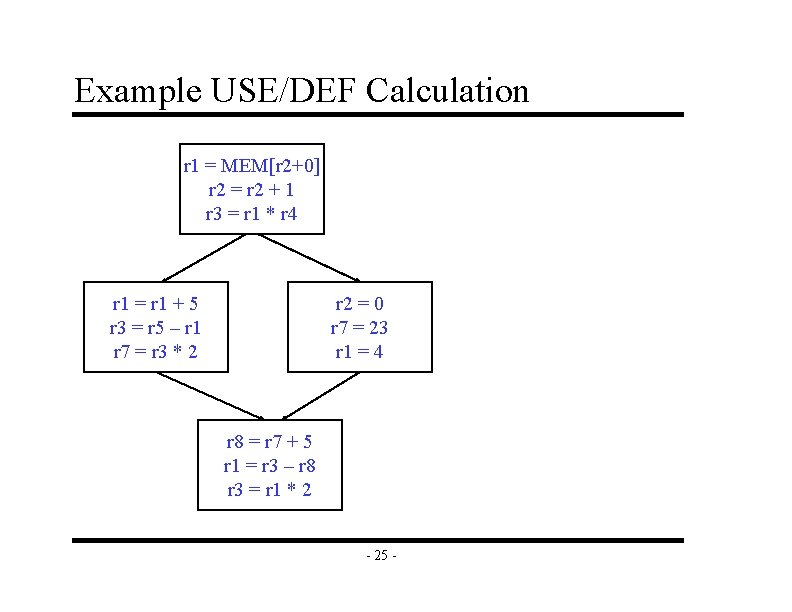Example USE/DEF Calculation r 1 = MEM[r 2+0] r 2 = r 2 + 1 r 3 = r 1 * r 4 r 1 = r 1 + 5 r 3 = r 5 – r 1 r 7 = r 3 * 2 r 2 = 0 r 7 = 23 r 1 = 4 r 8 = r 7 + 5 r 1 = r 3 – r 8 r 3 = r 1 * 2 - 25 -Compute IN/OUT Sets For All BBs initialize IN(X) to 0 for all basic blocks X change = 1 while (change) do change = 0 for each basic block in procedure, X, do old_IN = IN(X) OUT(X) = Union(IN(Y)) for all successors Y of X IN(X) = USE(X) + (OUT(X) – DEF(X)) if (old_IN != IN(X)) then change = 1 endif endfor IN = set of variables that are live when the BB is entered OUT = set of variables that are live when the BB is exited - 26 -Example IN/OUT Calculation r 1 = MEM[r 2+0] USE = r 2, r 4 r 2 = r 2 + 1 DEF = r 1, r 2, r 3 = r 1 * r 4 USE = r 1, r 5 DEF = r 1, r 3, r 7 r 1 = r 1 + 5 r 3 = r 5 – r 1 r 7 = r 3 * 2 r 2 = 0 r 7 = 23 r 1 = 4 r 8 = r 7 + 5 r 1 = r 3 – r 8 r 3 = r 1 * 2 - 27 - USE = DEF = r 1, r 2, r 7 USE = r 3, r 7 DEF = r 1, r 3, r 8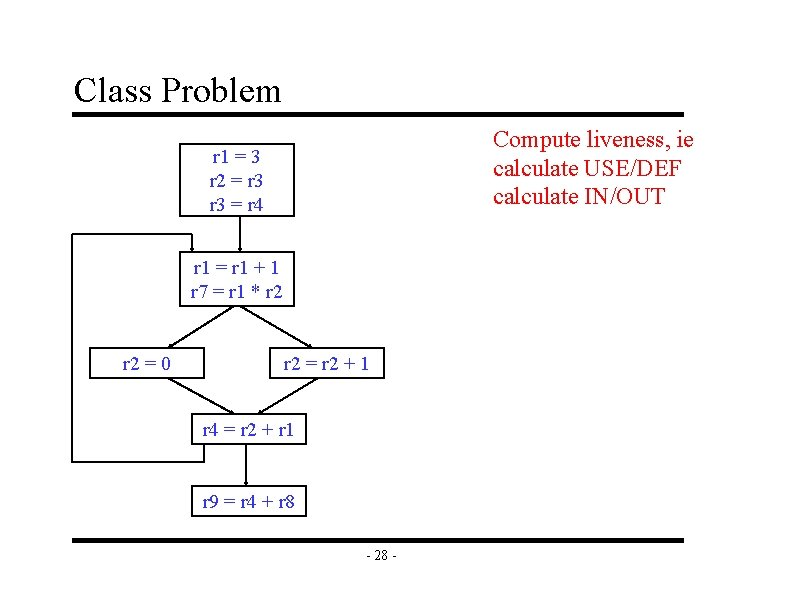Class Problem Compute liveness, ie calculate USE/DEF calculate IN/OUT r 1 = 3 r 2 = r 3 = r 4 r 1 = r 1 + 1 r 7 = r 1 * r 2 = 0 r 2 = r 2 + 1 r 4 = r 2 + r 1 r 9 = r 4 + r 8 - 28 -